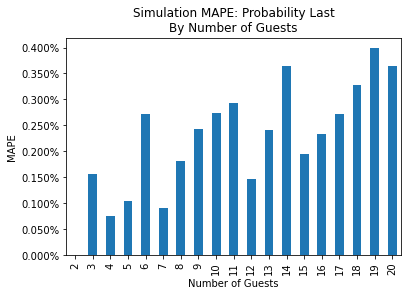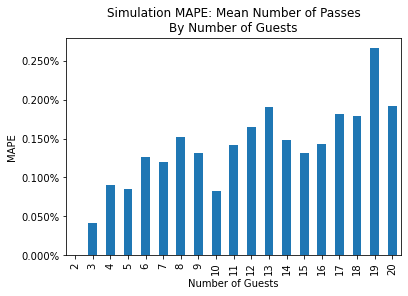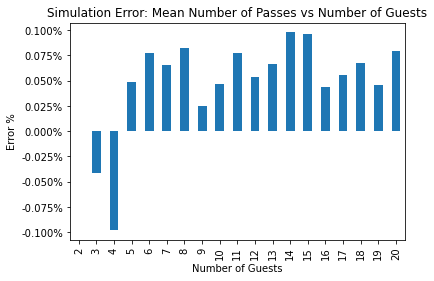In :
import os
import time

# math
import numpy as np
from numpy.random import default_rng, SeedSequence
import pathos.multiprocessing as mp

# data manipulation
import pandas as pd

# plotting
import matplotlib.pyplot as plt
import matplotlib.ticker as mtick

In :
def cranberry_passing_simulation(n_guests = 20, rng = default_rng()):
'''
Simulate cranberry passing process.

Inputs:
n_guests - number of guests at the table
rng - random number generator, uses default_rng by default (:
'''

# array whose i^th entry will be the i^th guest to receive
# the cranberry sauce

# initialize the problem
current_position = 0
n_passes = 0
pos = 

# iterate until everyone has received the cranberry sauce
step = 2 * rng.integers(0, 1, endpoint =True) - 1

current_position = (current_position + step) % n_guests
pos.append(current_position)
n_passes += 1


In :
def repeat_cranberry_passing_simulation(n_guests = 20, n_simulations = 10,
rng = default_rng()):
'''
Repeat the cranberry passing simulation.

Inputs:
n_guests - number of guests at the table
n_simulations - number of runs to simulate
rng - random number generator, uses default_rng by default (:
'''

# simulation results has an array with columns: received_last, n_passes
sim_rslts = np.zeros((n_simulations, 2), dtype = 'int')

# simulate
for i in range(n_simulations):
sim_rslts[i] = cranberry_passing_simulation(n_guests = n_guests, rng = rng)

sim_rslts = pd.DataFrame(sim_rslts, columns = ['received_last', 'n_passes'])

return sim_rslts

In :
def repeat_cranberry_passing_simulation_mp(n_guests = 20, n_simulations = 10, n_cores = 1,
seed = None):
'''
Multi-processing version of repeated cranberry passing simulation.

Inputs:
n_guests - number of guests at the table
n_simulations - number of runs to simulate
n_cores - number of multiprocessing threads to use
seed - random number generator seed, if none is provided, sets it randomly
'''

# produce a default seed if none ins provided
if seed is None:
seed = int(time.time())

# set up rng to run in parallel reproducibly if seed was provided
ss = SeedSequence(seed)
child_seeds = ss.spawn(n_cores)
streams = [default_rng(s) for s in child_seeds]

# simulations per core
n_sim_per_core = [n_simulations // n_cores for i in range(n_cores)]
n_sim_per_core = n_sim_per_core + (n_simulations % n_cores)

# perform the simulations on each core
with mp.Pool(n_cores) as p:
sim_rslts = p.map(
lambda x: repeat_cranberry_passing_simulation(n_guests = n_guests,
n_simulations = x,
rng = x),
zip(n_sim_per_core, streams)
)

# union all the result dataframes together
sim_rslts = (pd.concat(sim_rslts, axis='rows')
.reset_index()
.drop(columns = ['index']))

return sim_rslts

In :
%%time
import time

n_simulations = int(1e6)
n_guests_lo = 2
n_guests_hi = 20

for n_guests in range(n_guests_lo, n_guests_hi + 1):
tic = time.time()
sim_rslts = repeat_cranberry_passing_simulation_mp(n_guests=n_guests,
n_simulations = n_simulations,
n_cores = 16,
seed = 12345)

# compute proabilities and conditional mean number of passes
agg_rslts = agg_rslts.reset_index()
agg_rslts.columns = ['_'.join(col).strip() for col in agg_rslts.columns.values]
agg_rslts = agg_rslts.rename(
'n_passes_count' : 'count',
'n_passes_mean'  : 'mean_number_passes'}
)
agg_rslts['probability_last'] = agg_rslts['count'] / n_simulations
agg_rslts['n_guests'] = n_guests
agg_rslts = agg_rslts[['n_guests', 'guest_number', 'count', 'probability_last', 'mean_number_passes']]

# compute unconditional mean number of passes and inefficiency
agg_rslts_uncond_mean = pd.DataFrame(sim_rslts[['n_passes']].mean())
agg_rslts_uncond_mean.columns = ['mean_number_passes']
agg_rslts_uncond_mean = agg_rslts_uncond_mean.reset_index(drop=True)
agg_rslts_uncond_mean['n_guests'] = n_guests
agg_rslts_uncond_mean = agg_rslts_uncond_mean[['n_guests', 'mean_number_passes']]
agg_rslts_uncond_mean['inefficiency'] = (
agg_rslts_uncond_mean['mean_number_passes'] / (agg_rslts_uncond_mean['n_guests'] - 1) - 1
)

toc = time.time()
print("Simulation complete for n_guests = {} in {:0.02f} seconds.".format(n_guests, toc - tic))

if n_guests == n_guests_lo:
agg_rslts_all = agg_rslts
agg_rslts_uncond_mean_all = agg_rslts_uncond_mean
else:
agg_rslts_all = pd.concat([agg_rslts_all, agg_rslts])
agg_rslts_uncond_mean_all = pd.concat([agg_rslts_uncond_mean_all, agg_rslts_uncond_mean])

# finalize indices
agg_rslts_all = agg_rslts_all.reset_index(drop=True)
agg_rslts_uncond_mean_all = agg_rslts_uncond_mean_all.reset_index(drop=True)

Simulation complete for n_guests = 2 in 1.01 seconds.
Simulation complete for n_guests = 3 in 1.70 seconds.
Simulation complete for n_guests = 4 in 2.51 seconds.
Simulation complete for n_guests = 5 in 4.01 seconds.
Simulation complete for n_guests = 6 in 5.31 seconds.
Simulation complete for n_guests = 7 in 8.01 seconds.
Simulation complete for n_guests = 8 in 9.51 seconds.
Simulation complete for n_guests = 9 in 12.02 seconds.
Simulation complete for n_guests = 10 in 15.02 seconds.
Simulation complete for n_guests = 11 in 18.13 seconds.
Simulation complete for n_guests = 12 in 21.64 seconds.
Simulation complete for n_guests = 13 in 25.54 seconds.
Simulation complete for n_guests = 14 in 29.65 seconds.
Simulation complete for n_guests = 15 in 34.05 seconds.
Simulation complete for n_guests = 16 in 39.26 seconds.
Simulation complete for n_guests = 17 in 44.07 seconds.
Simulation complete for n_guests = 18 in 49.57 seconds.
Simulation complete for n_guests = 19 in 55.08 seconds.
Simulation complete for n_guests = 20 in 61.30 seconds.
CPU times: user 1.42 s, sys: 1.25 s, total: 2.67 s
Wall time: 7min 17s

In :
# export simulation results
agg_rslts_all.to_csv('./output/simulation_prob_and_mean_passes_last.csv', index=False)
agg_rslts_uncond_mean_all.to_csv('./output/simulation_mean_passes_and_inefficiency.csv', index=False)

In :
if os.path.exists('./output/analytical_prob_and_mean_passes_last.csv'):
df_compare_all = pd.merge(
agg_rslts_all,
agg_analytical_all,
on = ['n_guests', 'guest_number'],
how ='outer',
suffixes=['_sim', '_theory']
)

df_compare_all['mean_number_passes_pct_error'] = (
1 - df_compare_all['mean_number_passes_sim'] / df_compare_all['mean_number_passes_theory']
)
df_compare_all['probability_last_pct_error'] = (
1 - df_compare_all['probability_last_sim'] / df_compare_all['probability_last_theory']
)

# write comparison to file
df_compare_all.to_csv('./output/analytical_vs_sim_prob_and_mean_passes_last.csv')

# add temporarily for computing MAPE. drop when done
df_compare_all['abs_probability_last_pct_error'] = df_compare_all['probability_last_pct_error'].abs()
df_compare_all['abs_mean_number_passes_pct_error'] = df_compare_all['mean_number_passes_pct_error'].abs()

# compute MAPE
df_compare_all_mape = df_compare_all.groupby('n_guests')[
['abs_mean_number_passes_pct_error', 'abs_probability_last_pct_error']].mean()
df_compare_all_mape.rename(
columns = {'abs_probability_last_pct_error' : 'mape_probability_last',
'abs_mean_number_passes_pct_error' : 'mape_number_passes'},
inplace=True
)
df_compare_all_mape.reset_index(inplace=True)

# drop the temporary columns
df_compare_all.drop(columns = ['abs_mean_number_passes_pct_error', 'abs_probability_last_pct_error'],
inplace =True)

# Probability agreement with theory
fig, ax = plt.subplots(facecolor = 'w', figsize=(6,4))
df_compare_all_mape.plot.bar(x = 'n_guests', y = 'mape_probability_last', ax=ax, legend=False)
ax.set_title('Simulation MAPE: Probability Last\nBy Number of Guests')
ax.set_xlabel('Number of Guests')
ax.set_ylabel('MAPE')
ax.yaxis.set_major_formatter(mtick.PercentFormatter(1))

# Mean number of Passes aggreement with theory
fig, ax = plt.subplots(facecolor = 'w', figsize=(6,4))
df_compare_all_mape.plot.bar(x = 'n_guests', y = 'mape_number_passes', ax=ax, legend=False)
ax.set_title('Simulation MAPE: Mean Number of Passes\nBy Number of Guests')
ax.set_xlabel('Number of Guests')
ax.set_ylabel('MAPE')
ax.yaxis.set_major_formatter(mtick.PercentFormatter(1))In :
if os.path.exists('./output/analytical_mean_passes_and_inefficency.csv'):
df_compare_uncod_all = pd.merge(
agg_rslts_uncond_mean_all.drop(columns = ['inefficiency']),
agg_analytical_uncond_all.drop(columns = ['inefficiency']),
on = ['n_guests'],
how ='outer',
suffixes=['_sim', '_theory']
)
df_compare_uncod_all['mean_number_passes_pct_error'] = (
1 - df_compare_uncod_all['mean_number_passes_sim'] / df_compare_uncod_all['mean_number_passes_theory']
)

# write comparison to file
df_compare_all.to_csv('./output/analytical_vs_sim_mean_passes.csv')

# Mean number of Passes agreement with theory
fig, ax = plt.subplots(facecolor = 'w')
df_compare_uncod_all.plot.bar(x = 'n_guests', y = 'mean_number_passes_pct_error',
ax=ax, legend=False)
ax.set_title('Simulation Error: Mean Number of Passes vs Number of Guests')
ax.set_xlabel('Number of Guests')
ax.set_ylabel('Error %')
ax.yaxis.set_major_formatter(mtick.PercentFormatter(1))